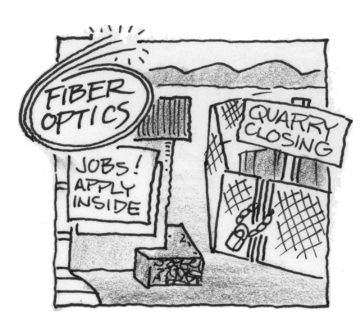### Home > APCALC > Chapter 6 > Lesson 6.2.2 > Problem6-76

6-76.

The good news for the small prairie town of Woisme is that a new fiber optics plant is about to open. The bad news is that the old quarry is about to close, and many residents will lose their jobs as a result. The predicted population over the next few years can be modeled by the following equation:

$p(t)=100(4·2^t-3^t)=$$400·2^t-100·3^t$

1. Using complete sentences, relate the equation for $p(t)$ to the situation.

There are both positive and negative components to $p(t)$. What do you think these components correspond to?

2. Algebraically determine when Woisme will have the greatest population. Use your calculator only for numerical calculations.

Find $p'\left(t\right)$ and determine when $p^\prime(t)=0$ .

Be sure to consider the endpoints. For example, Woisme's population might have been the greatest at the $t = 0$.

$≈ 2.283$ years